# Common Mode Voltage for Op Amp

#### Tony9168

Joined Mar 6, 2016
32

#### crutschow

Joined Mar 14, 2008
30,117
Does voltage follower has common mode voltage?
Of course.
Common-mode just means the voltages are essentially the same at both inputs.
Since the voltages at both inputs for the follower are the same, they are considered common-mode as far as the amp is concerned, since the amp doesn't know (or care) what is generating the two voltages.

This means the common-mode voltage varies with the signal amplitude for a follower amp.
It does not vary with signal amplitude for an inverting amp.

•Tony9168

#### Tony9168

Joined Mar 6, 2016
32
Hi Crutschow,

#### BR-549

Joined Sep 22, 2013
4,928

#### Tony9168

Joined Mar 6, 2016
32
Thanks for the video. It provides a very clear image for the common mode voltage.

#### Jony130

Joined Feb 17, 2009
5,351
Try again because your calculation is wrong. Also, do not forget that all voltage is measured with respect to ground.
And notice that your example does not show a stable situation. We have 2.5V at "-" input and 0V at "+" input.
So because of the fact that "-" > "+" the op-amp output will be at "negative saturation".

#### Tony9168

Joined Mar 6, 2016
32
Try again because your calculation is wrong. Also, do not forget that all voltage is measured with respect to ground.
And notice that your example does not show a stable situation. We have 2.5V at "-" input and 0V at "+" input.
So because of the fact that "-" > "+" the op-amp output will be at "negative saturation".
So because of the fact that "-" > "+" the op-amp output will be at "negative saturation".
"-" is the reference voltage (3.3V) of the inverting schmitt trigger while the voltage at "+" is 2.5V, it is lower than the reference voltage.
Therefore, the output of the inverting schmitt trigger should be 5V. (Supply is 5V)

Try again because your calculation is wrong. Also, do not forget that all voltage is measured with respect to ground.
I look through the circuit again but still unable to figure out which part of the calculation has error. Therefore, i explore the method of my calculation step by step and please point out which stage is wrong. Thanks.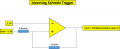Step 1: Calculate the UTP & LTP of the inverting schmitt trigger to determine its output when the input voltage is 2.5V.

From the calculation as shown below, the UTP & LTP after shifted by Vref is 3.334V & 3.266V. When the input voltage is 2.5V, the output will be 5V due to inverting characteristic.

Calculation:
Beta = (6.8k)/(6.8k+1M)= 17/2517
UTP = Beta x Vcc = 17/2517 ∗5V=0.034V
LTP = -Beta x Vcc = -17/2517 ∗5V=-0.034V

The UTP & LTP will be shifted by the reference voltage (3.3V), therefore the hysteresis of the inverting schmitt trigger is shown as below: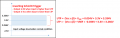Step 2: Determine the common mode voltage.

Common mode voltage = ((V+)+(V-))/2; (V-) = 2.5V while (V+) is unknown due to positive feedback circuit.
For easier visualisation, i have simplify the feedback circuit as shown below and through the calculation, the voltage at the (V+) pin is 0.015V.

Therefore, Vcm = (2.5V+0.0115V)/2 = 1.256V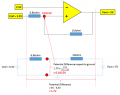#### Jony130

Joined Feb 17, 2009
5,351
"-" is the reference voltage (3.3V) of the inverting schmitt trigger while the voltage at "+" is 2.5V, it is lower than the reference voltage.
Therefore, the output of the inverting schmitt trigger should be 5V. (Supply is 5V)
Are we looking at the same diagram ??

Look hereIn this diagram (you draw it) I see 2.5V at "-" input and 0.0115V at the "+" input.
Do you see this or not ? Of cource you calculations about the voltage level at "+" input is wrong.

Step 1: Calculate the UTP & LTP of the inverting schmitt trigger to determine its output when the input voltage is 2.5V.
Here is my calculation

UTP - LTP = ΔVout * 6.8kΩ/1MΩ = 5V *6.8kΩ/1MΩ = 34mV

When the op-amp output voltage is 5V the voltage at the "+" input is equal to the 5V minus the voltage drop across 1MΩ resistor.

Hence:

UTP = (6.8kΩ*5V + 1MΩ*3.3V)/(6.8kΩ +1MΩ) = 3.31148V ≈ 3.31V

And to get LTP we need to find "+" input voltage when the op-amp output is at "negative saturation", 0V in your case.

LTP = 3.3V *1MΩ/(6.8kΩ +1MΩ) = 3.27771 ≈ 3.28V

Step 2: Determine the common mode voltage.

Common mode voltage = ((V+)+(V-))/2; (V-) = 2.5V while (V+) is unknown due to positive feedback circuit.
For easier visualisation, i have simplify the feedback circuit as shown below and through the calculation, the voltage at the (V+) pin is 0.015V.

Therefore, Vcm = (2.5V+0.0115V)/2 = 1.256V
Wrong again. First notice that the voltage at "-" input is 2.5V not 0.0115V.

Also did you ask yorself a question is yor answers has any sens?

If this 0.0115V was true the 1MΩ resistor current is I = (5V - 0.0115V)/1MΩ ≈ 5μA
The same current must flow through 6.8kΩ resistor hence the voltage drop across the 6.8kΩ resistor is 6.8kΩ*5μA ≈ 34mV and Vref needs to be lower than: 0.0115V - 0.034V = -0.0225 volts
As you can see something isn't right here.

The correct way of finding the voltage at "+" input is :
Because of the fact that Vo > Vref the current will flow in this direction Vo --->1MΩ--->6.8kΩ---->Vref.
And from KVL we can find V+ voltage

V+ = Vo - I1*1MΩ or
V+ = Vref + I1*6.8kΩ

Where

I1 = (Vo - Vref)/(1MΩ + 6.8kΩ) ≈ 1.69μA

And finally:

V+ = 5V - 1.69μA*1MΩ = 3.31V

And as a reminder, you should try to read this:

Last edited:
•Tony9168

#### Tony9168

Joined Mar 6, 2016
32
The correct way of finding the voltage at "+" input is :
Because of the fact that Vo > Vref the current will flow in this direction Vo --->1MΩ--->6.8kΩ---->Vref.
And from KVL we can find V+ voltage

V+ = Vo - I1*1MΩ or
V+ = Vref + I1*6.8kΩ
Appreciate and thanks for the example shown. I understand and realize my mistake.

The major mistake i made in the previous calculation is the calculated 0.0115V is not referring to the ground but referring to the 3.3V (Vref).

3.3V + 0.0115V = 3.3115V ≈ 3.31V.#### Tony9168

Joined Mar 6, 2016
32
1. Is CMRR important for a voltage follower?
From my perspective, it is not important as there is no differential voltage at the input pin of the voltage follower. Please advise if i get it wrong.

2. Is CMRR important for a integral amplifier?

Joined Mar 10, 2018
4,057
1) Yes -

https://www.analog.com/media/en/training-seminars/tutorials/mt-042.pdf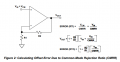If we let R2 go to 0, R1 to infinity, we are left with a generated offset of Vin / CMRR. Thats
because in a follower the feedback factor is < 1 because of finite Aol. In fact the G drops
over frequency aggravating the problem.

2) I would say no in most applications unless they are targeting high precision.

Regards, Dana.

Last edited:

#### Jony130

Joined Feb 17, 2009
5,351
Only the ideal op-amp the has a common-mode voltage gain (Acm) of zero. And the common-mode rejection ratio (CMRR), is defined as follows:
CMRR = Ad/Acm where Ad and Acm are the differential and common-mode gains respectively.

So for example, if you have the opamp with differential gain Ad = 100 000 V/V and CMRR = 85dB we can find common-mode gain Acm this way:

10^85/20 = 10^4.25 ≈ 17783

Acm = 100000/17783 = 5.6 V/V

And you can model CMRR this way: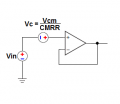As you can see you can model CMRR as DC offset voltage.

For Vin = 1V and CMRR = 85dB and Ad = 100 000V we have:

Vc = 1V/17783 = ± 56μV

And Vout_max = 1.00005V or Vout_min = 0.999934V becouse Vc can be equal to +56μV or -56μV.

This is exactly the same thing what Figure 2 from the danadak post was trying to tell.